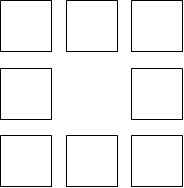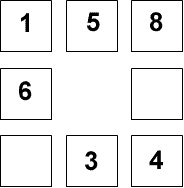#### You may also like### Prompt Cards

These two group activities use mathematical reasoning - one is numerical, one geometric.### Consecutive Numbers

An investigation involving adding and subtracting sets of consecutive numbers. Lots to find out, lots to explore.### Exploring Wild & Wonderful Number Patterns

EWWNP means Exploring Wild and Wonderful Number Patterns Created by Yourself! Investigate what happens if we create number patterns using some simple rules.

# Polo Square

##### Age 7 to 11Challenge Level

You may have seen Magic Squares before, where each row, each column and each diagonal adds to the same total. (Have a look at the problem called Magic Squares for an example.)

Here is a Polo Square:You can see that eight numbers could be arranged in the Polo Square - one in each box. In our Polo Square, the eight numbers can be chosen from the counting numbers $0$ to $9$ inclusive. A number cannot be used more than once. Each side of the Polo Square must add to the same total - we can call this the Polo total.

Here is a partly completed Polo Square:What is the Polo total?
Can you complete the Polo Square?

Can you find other ways of making a Polo Square with the same Polo total?

What other totals are possible?
Is there more than one way of making each one?

Many thanks to Alan Parr for this investigation. Alan has written several problem-solving maths adventures for 10 and 11 year-olds. The games are easy to use and very popular with children. Details can be obtained from Alan at alanparr@dial.pipex.com